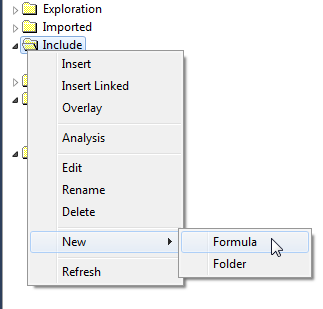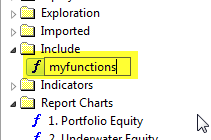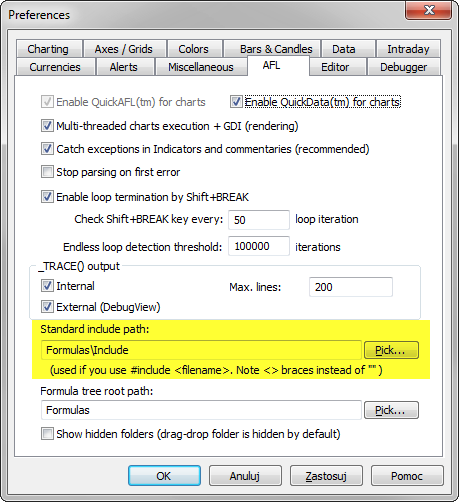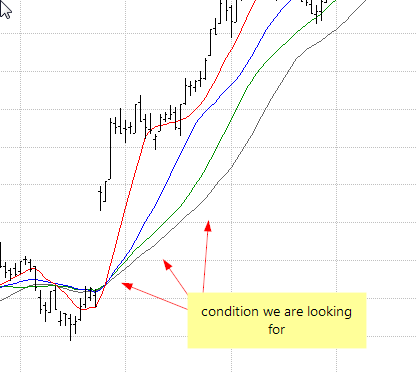#amibroker

### Calling custom user functions in our code

AFL language allows us to define reusable functions that can be used in our formulas. The following chapter of the manual explains the procedure in details: http://amibroker.com/guide/a_userfunctions.html

When we want to call such function in our formula, we should add function definition into our code, so AmiBroker could identify and interpret custom keyword properly. Consequently, if we use the function in multiple chart panes, each of the formulas should contain the function definition first.

`// custom function definitionfunction myMACD( array, fast, slow ){   return EMA( array, fast ) - EMA( array, slow );}// use of functionPlot( myMACD( High, 12, 26 ), "Open MACD", colorRed )`

Since we may potentially define a large group of our own functions, pasting the definitions manually may not be very convenient. To avoid that, we can use #include statement and group our definitions in a separate AFL file which will be called with a single statement from our main code.

To create such file we should do the following:

1. Create a new formula. The preferred location is in Include folder in chart windows, we can in fact choose any custom location of the file.2. We can also rename the file to a descriptive name, for example myfunctions.afl:3. Now we can edit the file and paste our function definitions, then save the file:
`function myMACD( array, fast, slow ){   return EMA( array, fast ) - EMA( array, slow );`
4. Now in our main file we can use only a reference to myfunctions.afl file:`// include our definitions#include <myfunctions.afl>// use of functionPlot( myMACD( High, 12, 26 ), "Open MACD", colorRed `

We don’t have to specify the path, because we saved our formula in the folder, which is specified as a ‘default include path’ in Tools–>Preferences–>AFL:In other cases we should provide full path to the file – #include is a pre-processor command, therefore this time we use single backslashes in the path:

#include “C:\Program Files\AmiBroker\AFL\common.afl”

http://www.amibroker.com/guide/afl/_include.html

### How to count symbols in given category

When we want to find out how many symbols belong to given category (such as watchlist) then for manual inspection, it is enough to hover the mouse cursor over the particular category name in the Symbols window and the information will be shown in a tooltip:If we want to check such information using AFL code, we could read the list of symbols returned with CategoryGetSymbols and by counting commas (which separate symbol names) find out the number of tickers.

A reusable function is presented below:

`function CategoryCountSymbols( category, number ){   count = StrCount( list = CategoryGetSymbols( category, number ), ",");   return IIf( list == "", 0, count + 1 );}    Title = "Symbols in watchlist 0: " + CategoryCountSymbols( categoryWatchlist, 0 )`

### How to fix Error 61 in printf/StrFormat calls

AmiBroker version 6.07 has introduced a strict check for correct string formatting in printf and StrFormat functions. These functions allow to specify the string followed by the list of arguments that will be inserted into the string in places, where %f, %g or %e specifiers are entered.

This works the following way:It is important for the list of subsequent arguments to match the number of % specifiers in the string. In cases, where there is no match – AmiBroker will display Error 61 message. Strict check is required because Microsoft C runtime crashes if wrong parameters are passed. Passing on earlier version was dangerous because it would lead to random crashes now and then depending on machine configuration.

There may be the following typical problems in the code:

Example 1. Four % specifiers, five value arguments

In this example formatting string contains four % specifiers so AmiBroker expects four arguments coming later, but five are given instead (too many).
`// WRONG - too many value argumentsTitle = StrFormat( "Open: %g, High: %g, Low: %g, Close: %g", Open, High, Low, Close, Volume );// CORRECTTitle = StrFormat( "Open: %g, High: %g, Low: %g, Close: %g", Open, High, Low, Close )`

Example 2. Five % specifiers, four value arguments

In this example formatting string contains five % specifiers so AmiBroker expects five arguments coming later, but four are given instead (too few).

`// WRONG - %.0f specifier does not have a matching argumentTitle = StrFormat( "Open: %g, High: %g, Low: %g, Close: %g, Volume: %.0f", Open, High, Low, Close );// CORRECTTitle = StrFormat( "Open: %g, High: %g, Low: %g, Close: %g, Volume: %.0f", Open, High, Low, Close, Volume )`

Example 3. Incorrectly coded percent (%) sign

In this example user wanted to print just % (percent sign), but used % (wrong) instead of %% (correct specifier of literal percent sign).

`// WRONG - to show the % sign in the output we need to use %%Title = StrFormat( "Close: %g (%.1f%)", Close, SelectedValue( ROC( Close, 1) ) );// CORRECT - you should use %% to print actual percent signTitle = StrFormat( "Close: %g (%.1f%%)", Close, SelectedValue( ROC( Close, 1) ) )`

The example 3 requires special attention, as it is a common mistake. Due to the fact that % sign is a special character, we need to use %% in our string if we want to print % sign in the output string.

### How to populate Matrix from a text file

AmiBroker 6.00 has introduced support for matrices. After we create a matrics with Matrix function call:

`my_var_name = Matrix( rows, cols, initvalue)`

then in order to access matrix elements, we need to use:
`x = my_var_name[ row ][ col ]`

However – if we want to populate a relatively large matrix with values generated in other programs, then it may not be very practical to do it by hand in the AFL code assigning individual elements like this:

`A[ 0 ][ 0 ] = 1; A[ 0 ][ 1 ] = 4; A[ 0 ][ 2 ] = 6`

What we can do in such case is to store the values in a text file that we could use as input, then read through the file using fgets function and populate Matrix elements using a looping code. A sample formula showing how to perform such task is presented below.

A sample text file for this example can be found here: http://www.amibroker.com/kb/wp-content/uploads/2015/10/samplematrix.txt

`// the input file pathfile = "C:\\samplematrix.txt";// define the size of the desired matrixrows = 16;cols = 16;// create matrixmyMatrix = Matrix( rows, cols, 0 );// open filefh = fopen( file, "r" );if( fh ){    i = 0;    // iterate through the lines of input file    for( i = 0; ! feof( fh ) AND i < rows; i++ )     {        // read a line of text        line = fgets( fh );         if( line == "" )        {            Error("Too few rows in the data file or an empty row found");            break;        }            // iterate through the elements of the line        for( j = 0; ( item = StrExtract( line, j ) ) != "" AND j < cols; j++ )         {            // assign matrix element            myMatrix[ i ][ j ] = StrToNum( item );        }                if( j < cols )        {            Error("Too few columns in data file");            break;        }    }        fclose( fh );}else{    Error( "ERROR: file can not be opened" );}// spot check selected elementTitle = "spot check M[ 2 ][ 3 ]: " + NumToStr( MyMatrix[ 2 ][ 3 ] )`

### Checking relationship between multiple moving averages

When we compare the positions of several lines against one another, we need to remember about using correct operators, so our AFL statement return correct results. Let us consider a set of moving averages using 10, 20, 30 and 40 periods, drawn with the following code:

`MA10 = MA( Close, 10 );MA20 = MA( Close, 20 );MA30 = MA( Close, 30 );MA40 = MA( Close, 40 );Plot( Close, "Close", colorDefault, styleBar );Plot( MA10, "MA10", colorRed );Plot( MA20, "MA10", colorBlue );Plot( MA30, "MA10", colorGreen );Plot( MA40, "MA10", colorgrey40 )`When we want to specify a condition where MA10 is highest of all of the lines, above MA20, which is above MA30 and with MA40 on the very bottom – we can NOT simply write:

`condition = MA10 > MA20 > MA30 > MA40; // WRONG - NOT what we really want, but no syntax erro`

It may seem strange that such statement is accepted without an error but it is actually syntactically correct. This is because of the fact that True and False are represented by numbers 1 and 0 respectively, so all comparisons actually have numerical value that allows such statement to be evaluated and yield numeric result. The above statement is evaluated from left to right and would be an equivalent of:

`condition = ( ( MA10 > MA20 ) > MA30 ) > MA40; // again WRON`

Using > operator will return an array of True or False values (1 or 0). Therefore – the result of MA10 > MA20 comparison (which is True, that is equal to 1) would be then compared to MA30, resulting in checking 1 > MA30, then if such condition returns False (i.e. 0 ), we would end up with 0 > MA40 comparison that would return False (0) as the final output. This is of course not what we want to get.

That is why we should use AND operator instead, because we want to check several conditions being met at the same time, that is:
`MA10 is above MA20AND // must use AND/OR to combine multiple conditionsMA20 is above MA30AND // must use AND/OR to combine multiple conditionsMA30 is above MA40`

Therefore, we should write the AFL statement the following way:

`condition = MA10 > MA20 AND MA20 > MA30 AND MA30 > MA40; // correct way of checking multiple condition`

So as a general guideline – if you have multiple boolean (yes/no) conditions that you want to combine into single rule, you need to use AND operator between conditions if you want True result when all conditions are met at the same time.

In a similar way, if you want a True result when any one (or more) of multiple conditions is met, then you need to use OR operator.

`condition = MA10 > MA20 OR MA20 > MA30; // this will give True result if any one condition (or both) is me`

### When and how often AFL code is executed?

All analysis in AmiBroker including charting, Analysis window or commentaries is based on underlying AFL code, which is being executed by the program to produce the required output. Therefore – any changes we see in the charts or analysis results (for example – chart updated with new ticks) mean that the program has received some input, then based on this information has recalculated the formula and presented the updated results.

These refreshes / formula recalculations depend on several factors:

1. In a local database, which is not updated by a realtime plugin, the formula would get refreshed if we perform any actions, such as clicking, scrolling, zooming, changing parameters, choosing another symbol or interval, importing new data etc.
2. In addition to the actions listed in (1), if we are running a plugin-based realtime feed, then the chart is refreshed based on “Intraday refresh interval” defined in Tools –> Preferences –> Intraday. If we enter 0 into this field, then it will result in chart being refreshed with every new tick (up to 10-times per sec).
3. It is also possible to force certain refresh rate using dedicated RequestTimedRefresh() function in the AFL code: http://www.amibroker.com/f?RequestTimedRefresh
4. It is also possible to manually refresh chart (or all chart and windows) using View->Refresh (or View->Refresh All) menu.

It is worth noting that chart formulas are refreshed only when they are placed on the active chart sheets. Non-active sheets just ‘don’t exist’, they are only created when you click on a bottom tab (sheet tab) to make them visible and destroyed immediately when other sheet becomes active. This ensures that precious CPU resources are not wasted on invisible chart sheets.

Additionally – by default charts in minimized chart windows or when multiple MDI windows are open and one is maximized, the windows in background that are completely obscured by others and/or minimized windows are not redrawn/refreshed during normal RT refresh. We can however call RequestTimedRefresh function with onlyvisible argument set to False and that will force regular refreshes in such windows as well.

`RequestTimedRefresh( 1 , False ); // force refresh for minimized MDI window or obscured MDI windo`

With regards to Analysis window – in general the formula is executed when we run e.g. Scan, Exploration, Backtest etc. Analysis window executes the formulas in multiple threads running in parallel (this tutorial explains multi-threading aspects: http://www.amibroker.com/guide/h_multithreading.html).

Repeated execution (to keep the code running over and over) in Analysis window can be also enabled with “Auto-repeat” option, the following Knowledge Base article explains it in details:

http://www.amibroker.com/kb/2014/10/03/how-to-setup-perodic-scans/

Last but definitely not least, we need to remember that AmiBroker may and will perform some executions internally for its own purposes such as:

1. during AFL Syntax Check that happens when applying the chart, or sending the code to Analysis window, or updating existing formula
2. when it is about to display Parameters window for the first time for given chart or during Parameters’ “Reset All” operation
3. at the beginning of Optimization when it reads Optimize() statements to configure the optimization process and/or smart optimization engines
4. at the beginning of each In-sample walk-forward step again to setup optimization parameters

Bottom line: we should never assume that certain formula will only be executed e.g. N-times during certain time-frame, because all really depends on the above factors, our actions and changing input.

### What are constants in AFL and how they work

The AFL language contains many pre-defined words like: shapeUpArrow, stopTypeTrailing, colorRed, styleThick, inDaily and many more. These are examples of constants. As written in AFL language specification (http://www.amibroker.com/guide/a_language.html): Constants are tokens representing fixed numeric or character values.

To better explain what this means, let us consider example of PI constant, which equals 3.14159265358979….. PI is the name of constant we use this name in mathematical equations, because it is easier and more practical to use than using the numerical value each time. Constants in AFL serve the same purpose, each of these words represents certain value properly interpreted by the program in the context they are used.

That is why using the following statement in backtesting code:

`ApplyStop( stopTypeTrailing, stopModePercent, 10 )`

is much better to use than cryptic statement like:

`ApplyStop( 2, 1, 10 )`

Both commands are equivalent, because value of stopTypeTrailing constant equals 2 and value of stopModePercent constant equals 1, yet the first version is much more understandable.

There is also another reason to use pre-defined constants rather than hard-coded numbers in the code. If for any reason the internal value of given constant changes due to development needs – all formulas using constants will continue to work properly (because new version would interpret them properly), while hard-coded numbers may change the code execution. For example – inWeekly and inMonthly constants have changed with introduction of N*inDaily timeframes, however if we always used:
TimeFrameSet( in Weekly ); in the code, then such internal change does not really affect our formulas at all.

There is one more example worth discussing – in the documentation of PlotShapes function we can find:

`Plot( Close, "Price", colorBlack, styleCandle );//Buy = Cross( MACD(), Signal() );Sell = Cross( Signal(), MACD() );//shape = Buy * shapeUpArrow + Sell * shapeDownArrow;//PlotShapes( shape, IIf( Buy, colorGreen, colorRed ), 0, IIf( Buy, Low, High ) )`

So – what does the multiplication mean in the above context? If we remember that constants are in fact just numbers, and boolean True in AFL has numeric value of 1, while boolean False has numeric value of 0, then:

– if Buy is True (equals 1) and Sell is False (equals 0), then the result of such calculation will be

`shape = 1 * shapeUpArrow + 0 * shapeDownArrow = shapeUpArrow`

– if Buy is False (equals 0) and Sell is True (equals 1), then the result of such calculation will be:

`shape = 0 * shapeUpArrow + 1 * shapeDownArrow = shapeDownArrow`

The above approach is kind of shortcut that saves using conditional statements. It would work correctly only if Buy and Sell signals never occur on the same bar and only if we assign just 0 or 1 (False / True) to Buy and Sell arrays. Otherwise the result of calculations would be different. The internal value of shapeUpArrow is 1 and ShapeDownArrow is 2, so in situation, where both Buy and Sell signals were true, we would get

`shape = 1 * shapeUpArrow + 1 * shapeDownArrow = shapeUpArrow + shapeDownArrow = 1 + 2 = 3`

So – we would then pass number 3 to PlotShapes function and this is neither shapeUpArrow nor ShapeDownArrow, but a different shape. That is why in general case it is better to use conditional function IIf, like shown below:

`shape = IIf( Buy, shapeUpArrow, IIf( Sell, shapeDownArrow, shapeNone ) )`

This way we are always sure that returned value will be shapeUpArrow or shapeDownArrow or shapeNone.

### Can I encrypt my formula so no-one can decipher it?

Currently the only way to protect your code from other peoples’ eyes is to translate part of the formula (such as few functions) or entire formula to C/C++ language and compile as AFL plugin DLL. Doing so requires some C/C++ programming knowledge, a C/C++ compiler (free Visual Studio Express or GNU tools can be used) and AmiBroker Development Kit (ADK).

ADK contains instructions how to write your own AFL plugin along with code samples that are ready-to-compile. Some C/C++ knowledge is required though.

or

NOTE: ADK is not for the beginners. It is for programmers (i.e. for people who already wrote some C/C++ code in their life). We are working on providing alternative methods for non-programmers.

### Do NOT make assumptions on number of bars

From time to time some users face “Error 10. Subscript out of range” in their formulas. The error itself is described in the manual, but still a few words of explanation why it happens may be useful.

The error usually occurs when formula uses hard-coded number of bars in the loop statements like this:

`for( i = 0; i < 300; i++ ) // MISTAKE: FIXED number of bars{  x = Close[ i ]; // ERROR 10. because 'i' becomes greater than BarCount`

or like this:

`for( i = BarCount - 1; i > BarCount - 300; i-- ) // MISTAKE: FIXED number of bars{  x = Close[ i ]; // ERROR 10. because 'i' becomes LESS than zero`

In both cases the code will FAIL if it is run on symbol that has LESS than 300 bars (BarCount < 300). In fact it will even fail on symbol that has more than 300 bars because of two facts:

1. during AFL Editor’s Verify Syntax not more than 200 most recent bars are used
2. a chart may be zoomed in so number of visible bars may be much lower and QuickAFL kicks in (so your AFL is executed with visible bars only).

This all means that one should never make any assumptions on number of bars your formula would get, because if you do, the formula will fail.

The formula should be written so it is able to execute without errors with BarCount as small as 1 (ONE).

This is normally done by writing a ‘for’ loop in a way recommended in the manual:

`for( i = 0; i < BarCount; i++ ){   x = Close[ i ]; // this will never produce Error 10 because i is in the range 0..BarCount-1`

If your formula references past data, say 10-bars earlier, you should start your loop with index 10, as below:

`for( i = 10; i < BarCount; i++ ){  x[ i ] = Close[ i ] - Close[ i - 10 ]; // both subscripts will be OK`

What to do if your formula, for some reason, really requires fixed number of bars? Well, the answer is that you should check if you really get as many bars as you think:

`if( BarCount > 300 ) // check first if you have enough bars{   // here we know that we have more than 300 bars   for( i = 0; i < 300; i++ )   {      x = Close[ i ];    }`

### A function with multiple return values

A typical AFL function returns one value. For example sin( x ) returns sine value of argument x. Sometimes however it is useful and/or required to return more than one value from the function.

Returning multiple values is possible only via arguments passed by reference, but trouble is that in AFL all arguments are passed by value (as in C language). Passing by value means that only value of variable is passed, not the variable itself, so original variable is not modified as shown in the example below:

`function Dummy( x ){    x = 7; // x is treated as function-local}//val = 10;Dummy( val );printf( "%g\\n", val ); // will print 10 because 'val' is unaffected by function cal`

The behaviour shown above is desirable because we usually want the function to be opaque and do not interfere with what is defined outside of the function except for returning the result of the function.

But what if we actually wanted to write to variables passed as arguments? Well that is possible if we pass the names of the variables as arguments.

`// This example shows how to return multiple values// the idea is to pass the name of the variable instead of// value//function fun_multiple_results( result1name, result2name ){  VarSet( result1name, 1 ); // setting variable using passed name  VarSet( result2name, 2 );  return;}//// to get multiple values from a function// we call the function passing NAMES of variables//a = 10;b = 20;printf("a = %g\\n", a );printf("b = %g\\n", b );//fun_multiple_results( "a", "b" ); // pass the names of variables//printf("a = %g\\n", a ); // see new values assigned to variablesprintf("b = %g\\n", b )`

Of course we can use arguments passed by name for two-way communication – we can use them as both inputs and outputs as shown in the following example that swaps the values of arguments

`function Swap( var1name, var2name ){    temp1 = VarGet( var1name ); // read the value from variable    temp2 = VarGet( var2name );    VarSet( var1name, temp2 ); // write the value to variable    VarSet( var2name, temp1 );}// Initial valuesx = 5;y = 37;//printf("Before swap x = %g, y = %g\\n", x, y );//Swap( "x", "y" ); // pass names of variables//printf("After swap x = %g, y = %g\\n", x, y )`

The code above will produce output like this:

Before swap x = 5, y = 37
After swap x = 37, y = 5

So it is clear that variables were passed to the function, swapped and returned successfully.

Next Page »